### Today: Excellent Function and Exemplary Formulas

In this lesson you will learn how to use TODAY function. This function is really easy and is more powerful than you thought.

The function returns the current system date, is mainly used in various forms. As well as in logistics, eg to calculate how many days was left to delivery / transportation day.

Syntax is just

=TODAY()

Excel will display current date eg. 2013-07-30

As you see above TODAY function has no arguments. Its correct result depends on correctly set the date (and time) in Windows.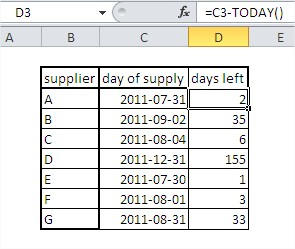### How to calculate how many years passed?

• Just use this formula: =YEAR(TODAY())-1986

Answer is 27 years. Yes, I am 27 years old.

• =INT((TODAY()-A1)/365.25) – write your date to A1 cell. It could we date from the future as well.
• YEAR(TODAY())-YEAR(A1) – another way to calculate year difference

### How to calculate time difference using TODAY function?

• =DATEVALUE(“2/11/2020”)-TODAY() – difference between your date and current date. Remember about proper formatting of cell. It must be GENERAL or NUMBER.
• =A1<TODAY()-10 – checks if A1 date is earlier than 10 days ago. It could be useful with checking some payments from clients. Displays TRUE or FALSE.
• =DATEDIF(A1,TODAY(),”y”) & ” Years, ” & DATEDIF(A1,TODAY(),”ym”) & ” Months” – displays how many years and months passed from A1 date and current date
• =DATEDIF(A1,TODAY(),”y”) & ” Years, ” & DATEDIF(A1,TODAY(),”ym”) & ” Months, ” & DATEDIF(A1,TODAY(),”md”) & ” Days” – gives you years, months and days between two dates

## Today function examples

### Simple today Formula

We need to find out today. There are different dates, and we could not find today’s date. We thought we do ourselves a favor, and just use the today’s formula.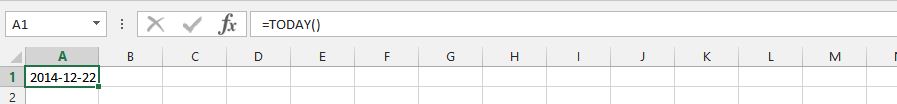### Today Plus 10 Days

We do have issues to find what the date would be ten days from now. This is critical, and we need to find out the today’s formula to find the date for that day.### Crazy Date’s Calculation

We are trying to find out the date, based on different assessments that would allow us to add today with a week ago. This is why we are using the today’s formula to find the appropriate answer.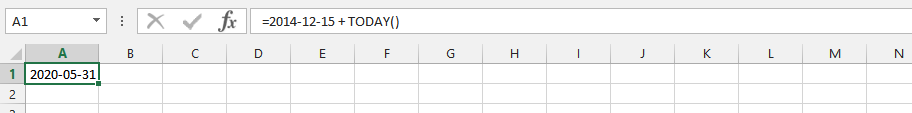### Date Value and Today Formula

The situation is that we would like to find out the numbers of days between today and certain date in the future. A situation like this make it important to use both the date values and today formulas to get the result. It is also critical to format cell to number, because it would return with the total numbers of days.### DAY and TODAY

We just want to know today’s number in the month. It is not about finding out the date, but about today.### Month and Today

We would like to find out the current month of the day. This is why it is important to use both month and today formula simultaneously.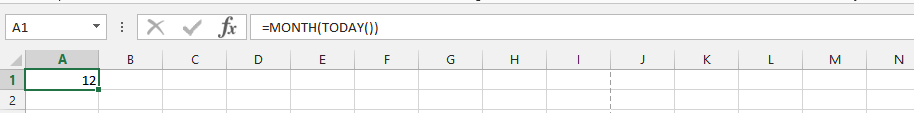### Year and Today Formula

The circumstances is quite different when the information we would need is specific. This is because we would like to know the age of the person, who is celebrated his birthday today. It would help to write the birthday card.### Double Today Formula

We are trying to find out the process, and we want to out the process time. This is why we are using the double today’s formula.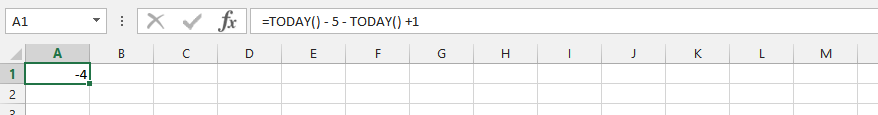### Double Today and Month Formula

It is time to find out the period between the today and month formula in the process.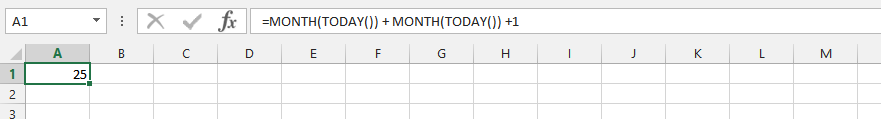### Double Year and Today

We want to find out if we could use the year and today formula to find out the numbers of days within the period.## Other examples of TODAY function

• =DAY(TODAY()) – extracts current day
• =MONTH(TODAY()) – extracts current month
• =WEEKDAY(TODAY()) – extracts number of the day of the week
• =TEXT(WEEKDAY(TODAY()),”dddd”) – displays name of the day of the week
• =DATE(YEAR(TODAY()),MONTH(TODAY())+1,1)-1 – displays the last day of current month
• =IF(TODAY()=A1,TRUE,FALSE) – checks if A1 is current date and displays TRUE or FALSE
Previous articleHow To Link Objects In Excel